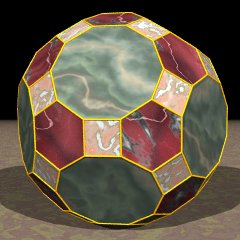Acronym grid
TOCID symbol tID
Name great rhombicosidodecahedron (but not qrid),
truncated icosidodecahedron,
omnitruncated icosahedron,
omnitruncated dodecahedron

`© ©`
Vertex figure [4,6,10]
Vertex layers
 Layer Symmetry Subsymmetries o3o5o o3o . o . o . o5o 1 x3x5x x3x .{6} first x . x{4} first . x5x{10} first 2 x3F . u . F . u5x 3 u3U . x . C . x5F 4a x3A . X . U . x5U 4b X3F . 5 B3x . F . A . u5F 6 F3C . B . F . X5x 7 D3x . Y . x . x5X 8 U3C . U . D . F5u 9 D3F . D . X . U5x 10a F3D . H . u . F5x 10b A . B 11 C3U . F . H . x5u 12a x3D . J . x . x5xopposite {10} 12b C . Y 12c x . J 13a C3F . J . x 13b C . Y 13c x . J 14 x3B . F . H 15a A3x . H . u 15b F3X . A . B 16 U3u . D . X 17 F3x . U . D 18 x3x .opposite {6} Y . x 19 B . F 20 F . A 21 X . U 22 x . C 23 u . F 24 x . xopposite {4}
(F=x+f, U=2f, X=2x+f, A=3f, B=2x+2f, C=x+2f, D=x+3f, Y=3x+2f, H=2x+3f, J=4f)
General of army (is itself convex)
Colonel of regiment (is itself locally convex – no other uniform polyhedral members)
Dihedral angles
• between {4} and {6}:   arccos(-(1+sqrt(5))/sqrt(12)) = 159.094843°
• between {4} and {10}:   arccos(-sqrt[(5+sqrt(5))/10]) = 148.282526°
• between {6} and {10}:   arccos(-sqrt[(5+2 sqrt(5))/15]) = 142.622632°
Confer
variations:
a3b5c   f3x5x   v3x5f   f3v5v   x3(-x)5f
ExternalAs abstract polytope grid is isomorphic to gaquatid, thereby replacing decagons by decagrams.

Incidence matrix according to Dynkin symbol

```x3x5x

. . . | 120 |  1  1  1 |  1  1  1
------+-----+----------+---------
x . . |   2 | 60  *  * |  1  1  0
. x . |   2 |  * 60  * |  1  0  1
. . x |   2 |  *  * 60 |  0  1  1
------+-----+----------+---------
x3x . |   6 |  3  3  0 | 20  *  *
x . x |   4 |  2  0  2 |  * 30  *
. x5x |  10 |  0  5  5 |  *  * 12

snubbed forms: β3x5x, x3β5x, x3x5β, β3β5x, β3x5β, x3β5β, s3s5s, β3β5β
```